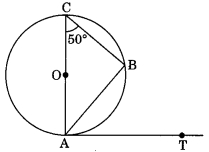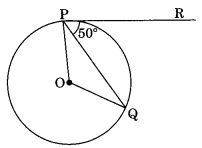CBSE Class 10 Circles MCQ New Pattern

## CBSE Class 10 Circles MCQ New Pattern By Clarify Knowledge

CBSE Class 10 Circles MCQ New Pattern 2022

## CBSE Class 10 Circles MCQ Table

Question 1.
If radii of two concentric circles are 4 cm and 5 cm, then the length of each chord of one circle which is tangent to the other circle is
(a) 3 cm
(b) 6 cm
(c) 9 cm
(d) 1 cm

Question 2.
In Fig., if ∠AOB = 125°, then ∠COD is equal to(a) 62.5°
(b) 45°
(c) 35°

Question 3.
If Fig., AB is a chord of the circle and AOC is its diameter such that ∠ACB = 50°. If AT is the tangent to the circle at the point A, the ∠BAT is equal to(a) 65°
(b) 60°
(c) 50°

Question 4.
From a point P which is at a distance of 13 cm from the point O of a circle of radius 5 cm, the pair of tangents PQ and PR to the circle are drawn. Then the area of the quadrilateral PQOR is
(a) 60 cm²
(b) 65 cm²
(c) 30 cm²

Question 5.
At one end A of a diameter AB of a circle of radius 5 cm, tangent XAY is drawn to the circle. The length of the chord CD parallel to XY and at a distance 8 cm from A is
(a) 4 cm
(b) 5 cm
(c) 6 cm

Question 6.
In Fig., AT is a tangent to the circle with centre O such that OT = 4 cm and ∠OTA = 30°. Then AT is equal to(a) 4 cm
(b) 2 cm
(c) 2√3 cm

Question 7.
In Fig., if O is the centre of a circle PQ is a chord and the tangent PR at P makes an angle of 50° with PQ, then ∠POQ is equal to(a) 100°
(b) 80°
(c) 90°

Question 8.
In Fig., if PA and PB are tangents to the circle with centre 0 such that ∠APB = 50°, then ∠AOB is equal to(a) 25°
(b) 130°
(c) 100°

Question 9.
If two tangents inclined at an angle 60° are drawn to a circle of radius 3 cm the length of each tangent is equal to
(a) 32√3 cm
(b) 6 cm
(c) 3 cm